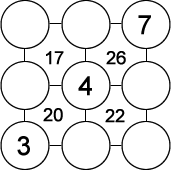Select Page

The number in each square represents the sum of the numbers in the four surrounding circles.  The numbers in the circles can only be 1 to 9 and each number can be used only once.  Three numbers have been provided to get you started.  Find the remaining six numbers.[donotprint]

### Solution### Solution explanationComments on solving the puzzle; a process of eliminating possible values as answers:

Values 3, 4 & 7 are provided and can not be reused.

Starting at Equation (1), unknowns B & D can only be equal to 6 or 9 as shown. All other combinations include either a 3, 4 or 7 value.

Equation (2): unknowns D, F & E can only be equal to 1, 8 or 9 as shown since values 3, 4 & 7 can not be reused. Therefore comparing with Equation (1), D can only be equal to 9 and E & F can be equal to 1 or 8. Comparing with Equation (1), B is then equal to 6.

Equation (3): unknowns C & E can be equal 5 or 8 as shown. Comparing with Equation (2), E can only be equal to 8 & C is then equal to 5.

Equation (4): unknowns A, B & C can only be equal to 2, 5 or 6 as shown. Therefore comparing with Equation (1), (2) & (3) with B equal to 6, C is then equal to 5 and A is equal to 2.

Now insert your final answers into the original puzzle and verify the resulting sums.

## Feedback

There are more than one way of doing these puzzles and may well be more than one answer.  Please let me and others know what alternatives you find by commenting below.  We also welcome general comments on the subject and any feedback you’d like to give.   If you have a question that need a response from me or you would like to contact me privately, please use the contact form.[/donotprint]

## Get more puzzles!

If you’ve enjoyed doing the puzzles, consider ordering the book; 150+ of the best puzzles in a handy pocket sized format. [donotprint]Click here for full details.[/donotprint]